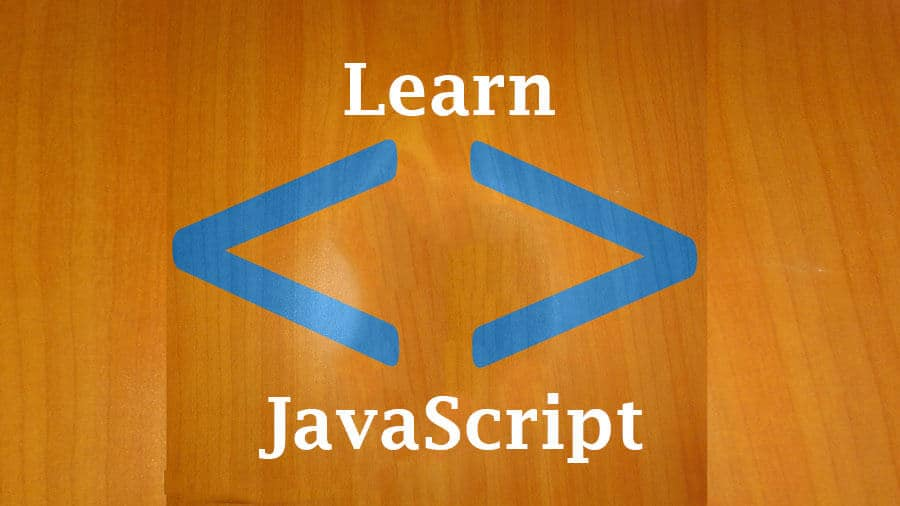# 38 Integer Literals octal numbers – JavascriptLast updated on June 30th, 2018 at 08:49 pm

This video is taken from the full course that will teach you HTML, CSS, Programming concepts and Javascript .

Transcript :

Hi, and welcome back.

So in the last video, we so how you can represent a hexadecimal number within your code.

So in this video, we’re going to look at binary numbers and how you can represent a binary number within your code.

So if at any point you want to use a binary number, it will start with zero and ‘b’ for binary or zero and a capital ‘B’ for binary still.

Even here, the ‘o’ is for octal and here the ‘x’ is for hexadecimal.

If you want to tell the system right away that the number that we’re talking about right now, let me remove all of this, remove everything.

You want to represent a number as a binary, you want to let the system know that a numbers is a binary, you’ll just do ‘0b’ and whatever number it is, 10 or maybe 10.

Then you display that is 10.

Then let’s say we declare a variable, we’ll call it num=0b1010.

Then now we can do different things with our variable.

Let’s say num, num*num.

What result will it give us? 100.

We can even change it to 000001.

Then we display which number.

This is, you can see, we can also display the value of ‘num’ so that you can know which number we are working with.

So if I display, you see this is a number that this is equivalent to.

In binary numbers you know it only has zero and one.

So at any point within this if I have something like 2, we know this is going to give us an error.

It’s going to be an error because ‘2’ is not part.

Because we know that 2 is not part of the possible numbers in a binary number.

If I display this, you see it gives us all these errors.

If you want the system to know that the number you’re using is a binary number, it will start with zero and a small ‘b’ or a capital ‘B’ and the numbers within it must be a ‘0’ or a ‘1’.

So I hope by now you understand the different kind of integer literals that you can use.

You can use a number as a decimal, you can use as a octal, you can use as a hexadecimal, you can use as a binary.

And you know how you can represent a numbers as an octal.

That is using zero and ‘o’ or zero and capital ‘O’ and as a hexadecimal by using zero and ‘x’ or zero and capital ‘X’ and as a binary buy using zero and small ‘b’ or zero and capital ‘B’.

And as I said in most cases, you’re only going to find yourself using decimal numbers.

And with decimal numbers, they’re very easy to write.

If you want to write 24, 24.

No, nothing in front of it.

You want to write 30, just that simple.

You want to write 2030 you don’t have to use anything before it or after it.

As long as it’s a number that contain 0-9 with nothing in front of it, it will definitely automatically be interpreted as a decimal number.

So that’s it for this section on integer literals and I will see you in the next section.

If you have any questions, feel very free to let me know.

So I’ll see you in the next section.

Click the button below to watch this full course: